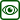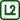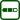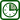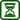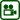## Thermal Volumetric Expansion of Metals

### Experiment number : 1950

• #### Goal of experiment

This experiment demonstrates the increase of volume of a metal ball when it is heated.

• #### Theory

Similarly to liquids and gases, the volume of solids is also dependent on their current temperature. Most solids increase their volume with increasing temperature, and this increase in volume ΔV is (with some negligence) directly proportional to an increase in temperature Δt and the initial volume V0. This relation can be mathematically described as

$\Delta V\,\doteq\,\beta V_0 \Delta t,\tag{1}$

where the constant β is the volumetric thermal expansion coefficient, a property of substances. In the tables we usually find for solids their linear thermal expansion coefficient α, and it approximately holds for isotropic substances that $$\beta\,\doteq\,3\alpha$$. Volume V after heating is then equal to the initial volume V0 and the increase in volume ΔV given with the relation (1):

$V\,\doteq\,V_0\,+\,\beta V_0 \Delta t\,=\,V_0(1\,+\,\beta\Delta t). \tag{2}$

The negligence described above limits the validity of relation (2) to “small” temperature differences where βΔt≪1. If this condition is not sufficiently met, we need to use the relation (2) in its more general form:

$V\,\doteq\,V_0(1\,+\,\beta\Delta t\,+\,\frac{1}{3}\beta^2\Delta t^2). \tag{3}$

In the following experiment we are going to see the increase in volume of a metal ball after heating it to a few hundred degrees Celsius.

• #### Tools

Metal ball with a metal hinge and a hoop through which the ball can barely fit at room temperature, gas burner, matches, pliers.

• #### Procedure

Basic execution of the experiment

1. We show that at room temperature the ball freely moves through the hoop.

2. We light the gas burner and let the ball heat inside the flame for a few seconds to tens of seconds.

3. We turn off the burner and put the ball on the hoop – now it does not go through.

4. We wait until the ball cools down enough to again fall through the hoop.

Expanding the experiment to a problem task

1. We can directly follow point 4 – when the ball falls through the hoop, we can try to lift it back up again.

2. We find out that during the lifting the ball gets “stuck” again; this is repeated when we again put the ball on the hoop (see video in part Sample result). Explanation of this phenomenon is given in the section Pedagogical notes.

• #### Sample result

The course of both the basic and the extended version of the experiment is shown in the video below.

• #### Technical notes

• The metal hinge of the ball can be a very good thermal conductor, which is why it is advisable to use pliers during its manipulation.

• As a hinge we use a chain or a wire that will not burn after putting the ball into the flame.

• We do not recommend heating the ball with a candle – the process is slow and the ball is covered with a layer of soot.

• Safety warning: The ball reaches several hundred degrees Celsius during the experiment and great care must be taken when working with it! We must especially avoid dropping the ball on a material that could melt upon contact!

• #### Pedagogical notes

• It is important for illustrative purposes not to leave out point 1 where the ball is still at room temperature.

• If the ball is insufficiently heated, it will fall through the hoop similarly to when it was at room temperature; on the other hand, if we heat it too much, we can wait for several minutes until it falls through again. The optimal rate of heating depends mostly on the heat source used; that is why it is necessary to try the experiment beforehand and estimate the correct time of heating.

• If we do not want to introduce the problem part into the experiment, we can simply show the basic version.

• The extended execution of the experiment gives us a result that students usually do not anticipate. Especially if after the first fall we suggestively ask “Will the ball come back up through the hoop?”, students are very surprised that it is not so. The explanation lies in the fact that the hoop heats up from the ball and therefore also expands. When the ball falls through, the hoop starts to cool down again, much faster than the ball. When we try to pull the ball back up, we hit the shrinking hoop, which causes the ball to get stuck again. This “getting stuck” repeats in both directions until the temperatures of the ball, the hoop and the surrounding air become close enough.

• #### Link to a related problem

To solve a similar problem, see Thermal expansion of brass balls.